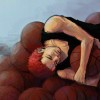[D] [A] [Bm7] [G] [A7] [D]整晚胡思[A]乱想 夜[Bm7]色真好 [G]让我睡不着 [D]为何你总[A7]是想要[G]逃 [D]相思若[A]好不了 只[Bm7]能怪我[A]找不到解[D]药 [D]你从未[A7]给过我爱的[G]讯号 糟[D]糕 我[A7]陷得比你[Bm7]早 你[A]爱的比我[D]少 注定[A]要受煎[G]熬 不[D]好 优[A7]雅都不见[Bm7]了 不[A]安分的心[D]跳 全世[A]界都听[G]到 [G]别那么骄傲 [D]我随时可[A]能走掉 [D]我的手你[G]还没有牵到 [D]夜太长[A]月光必定[G]会冷掉 [Bm7]如何是好 [D]你欠我[A]一个拥抱 [D]而我却一[G]再对你微笑 [D]怎么你[A]还没看见[G]我的好 [D]整晚胡思[A]乱想 夜[Bm7]色真好 [G]让我睡不着 [D]为何你总[A7]是想要[G]逃 [D]相思若[A]好不了 只[Bm7]能怪我[A]找不到解[D]药 [D]你从未[A7]给过我爱的[G]讯号 糟[D]糕 我[A7]陷得比你[Bm7]早 你[A]爱的比我[D]少 注定[A]要受煎[G]熬 [G]别那么骄傲 [D]我随时可[A]能走掉 [D]我的手你[G]还没有牵到 [D]夜太长[A]月光必定[G]会冷掉 [Bm7]如何是好 [D]你欠我[A]一个拥抱 [D]而我却一[G]再对你微笑 [D]怎么你[A]还没看见[G]我的好 [G]别那么骄傲 [D]我随时可[A]能走掉 [D]我的手你[G]还没有牵到 [D]夜太长[A]月光必定[G]会冷掉 [Bm7]如何是好 [D]你欠我[A]一个拥抱 [D]而我却一[G]再对你微笑 [D]抱着我[A]的苦恼睡[G]着了觉 [G]别那么骄傲 D]我随时可[A]能走掉 [D]我的手你[G]还没有牵到 [D]夜太长[A]月光必定[G]会冷掉 [Bm7]如何是好 [D]你欠我[A]一个拥抱 [D]而我却一[G]再对你微笑 [D]抱着我[A]的苦恼睡[G]着了觉 [G]别那么骄傲 D]我随时可[A]能走掉 [D]我的手你[G]还没有牵到 [D]夜太长[A]月光必定[G]会冷掉 [Bm7]如何是好 [D]你欠我[A]一个拥抱 [D]而我却一[G]再对你微笑 {title:那么骄傲} {artist:金海心} {author:刘小寒}